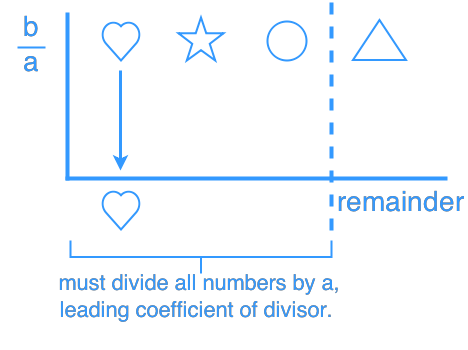# Polynomial synthetic division - Polynomial Functions

### Polynomial synthetic division

Synthetic division is a shortcut method of dividing polynomials as opposed to long division. Yet, this method can only be used when we are dividing a liner expression and the leading coefficient is a 1.

#### Lessons

###### Synthetic division by $\left( {x - b} \right)$